Ex 1.4

Chapter 1 Class 11 Sets
Serial order wise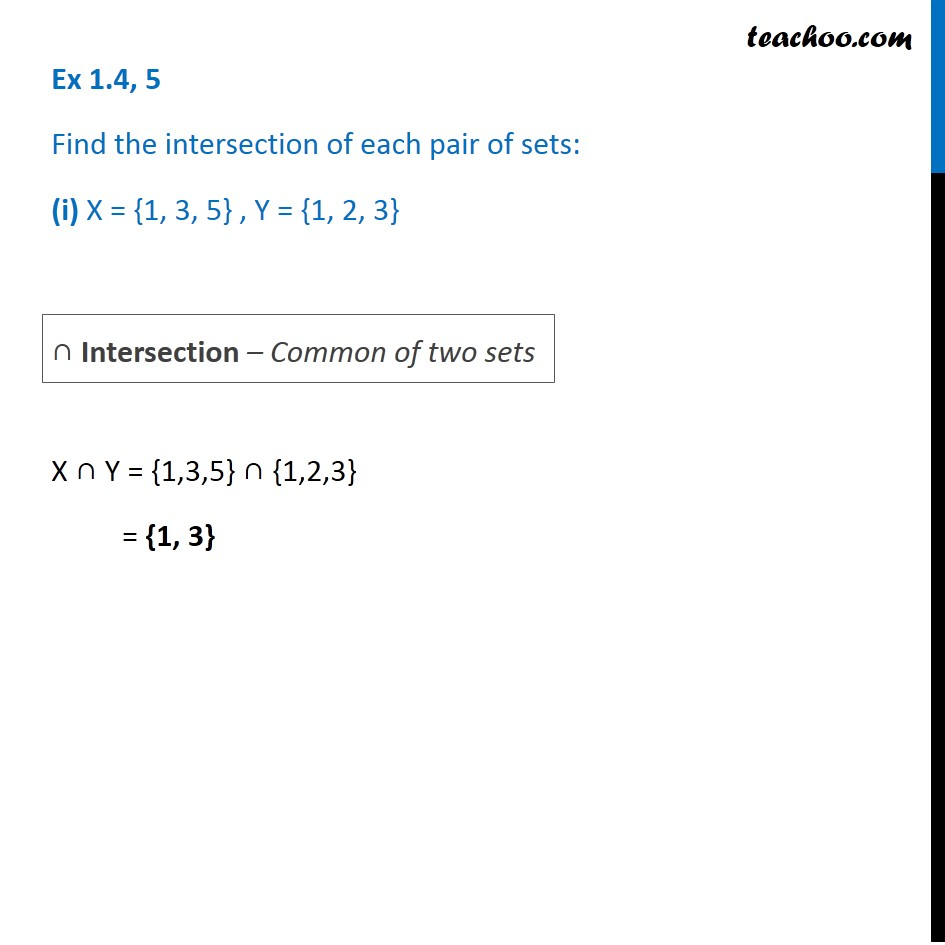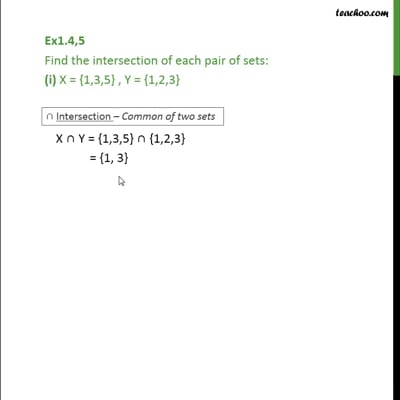This video is only available for Teachoo black users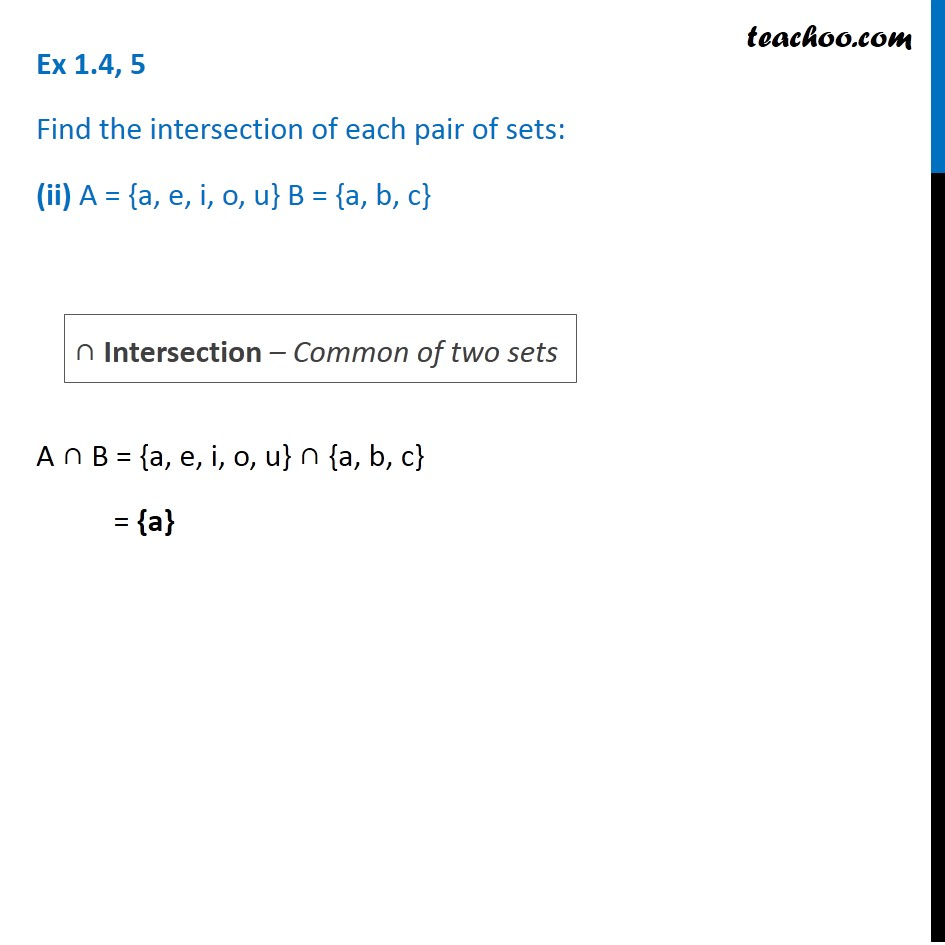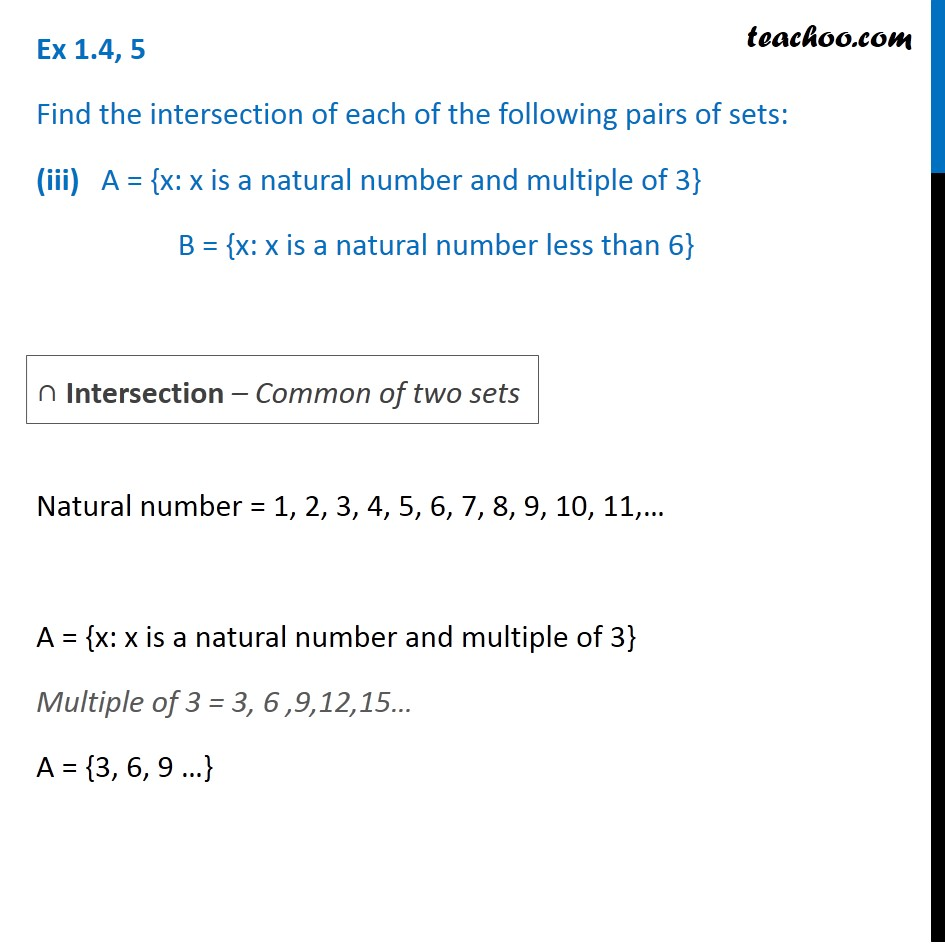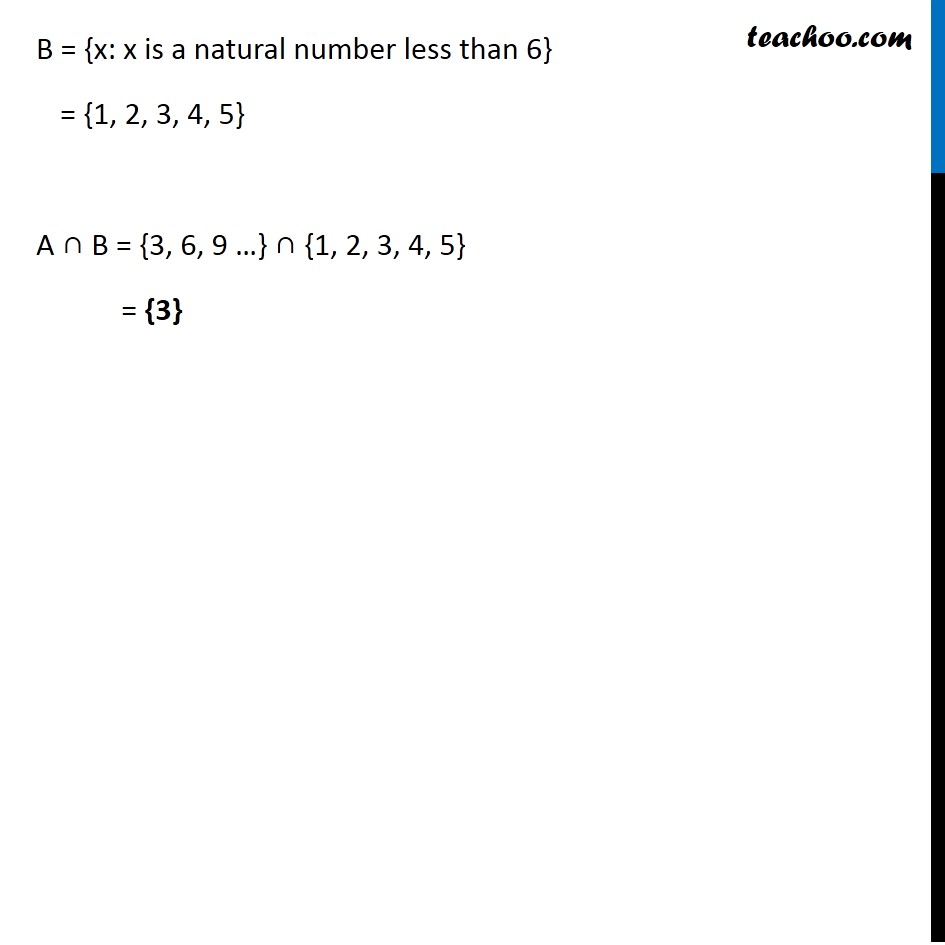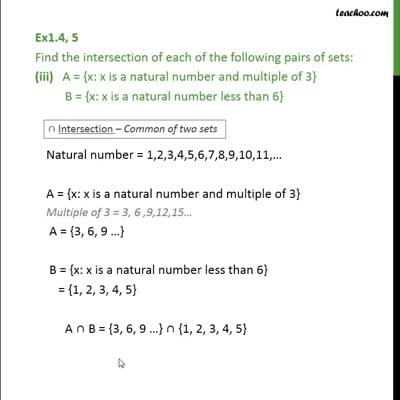This video is only available for Teachoo black users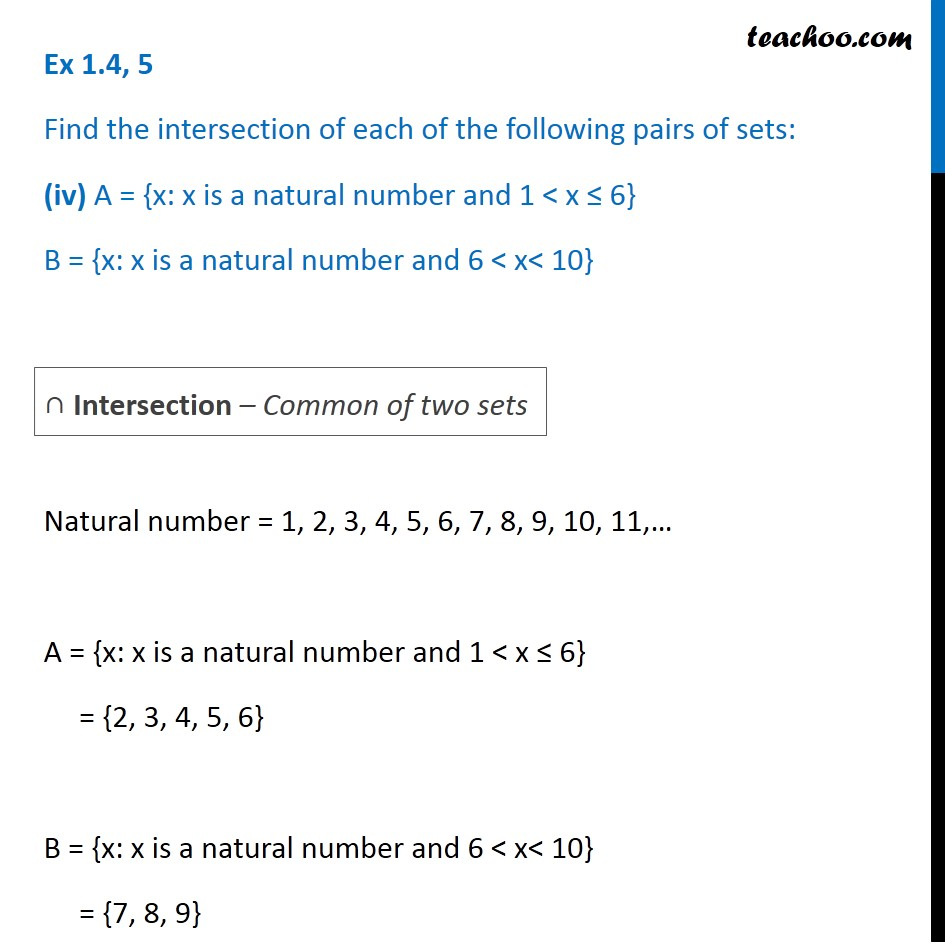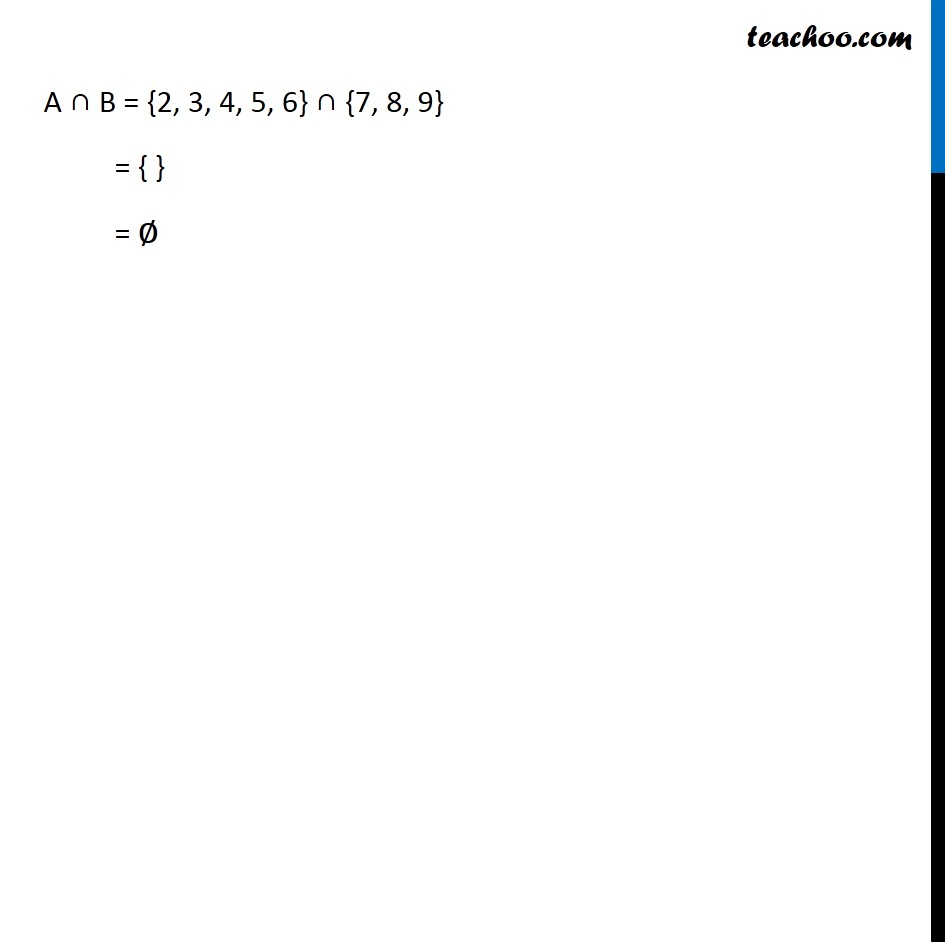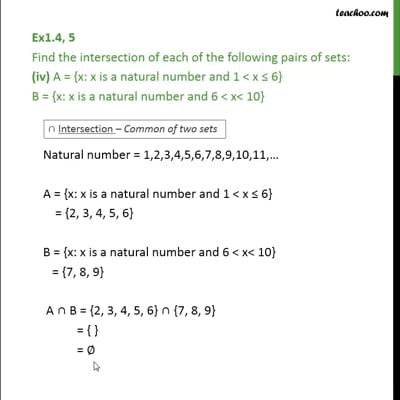This video is only available for Teachoo black users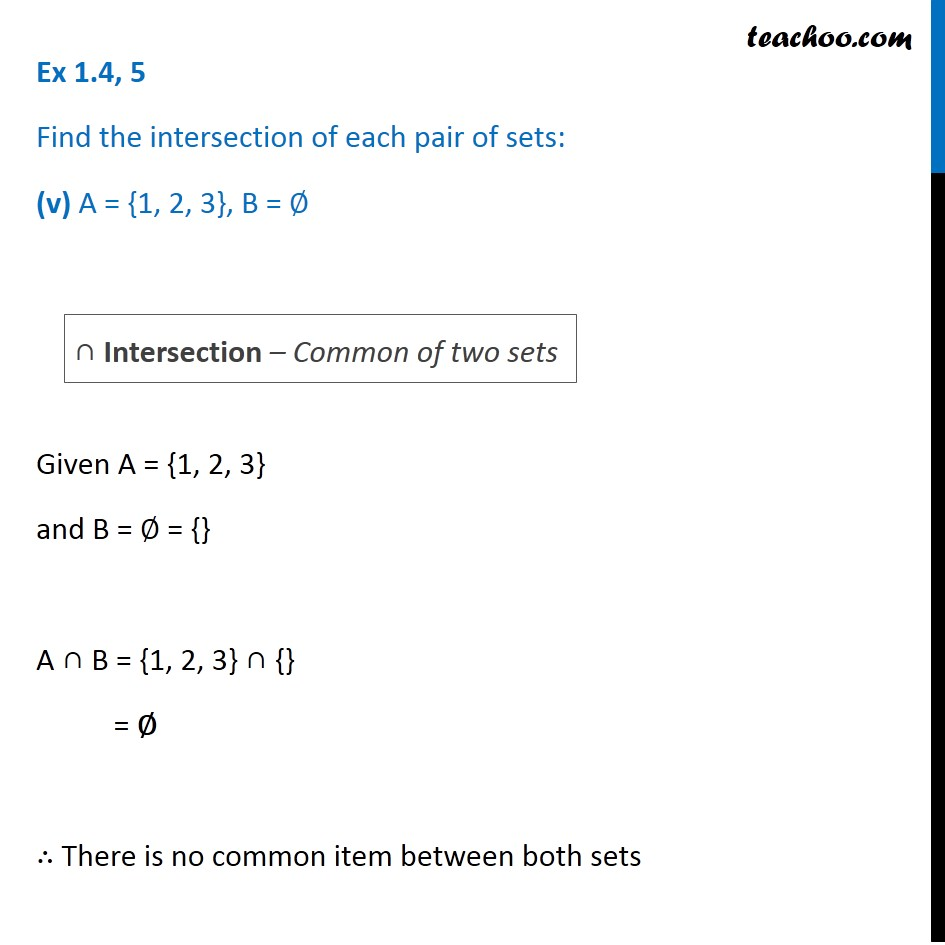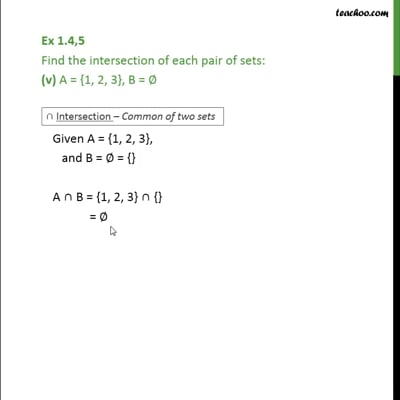This video is only available for Teachoo black users

Solve all your doubts with Teachoo Black (new monthly pack available now!)

### Transcript

Ex 1.4, 5 Find the intersection of each pair of sets: (i) X = {1, 3, 5} , Y = {1, 2, 3} X ∩ Y = {1,3,5} ∩ {1,2,3} = {1, 3} ∩ Intersection – Common of two sets Ex 1.4, 5 Find the intersection of each pair of sets: (ii) A = {a, e, i, o, u} B = {a, b, c} A ∩ B = {a, e, i, o, u} ∩ {a, b, c} = {a} ∩ Intersection – Common of two sets Ex 1.4, 5 Find the intersection of each of the following pairs of sets: (iii) A = {x: x is a natural number and multiple of 3} B = {x: x is a natural number less than 6} Natural number = 1, 2, 3, 4, 5, 6, 7, 8, 9, 10, 11,… A = {x: x is a natural number and multiple of 3} Multiple of 3 = 3, 6 ,9,12,15… A = {3, 6, 9 …} ∩ Intersection – Common of two sets B = {x: x is a natural number less than 6} = {1, 2, 3, 4, 5} A ∩ B = {3, 6, 9 …} ∩ {1, 2, 3, 4, 5} = {3} Ex 1.4, 5 Find the intersection of each of the following pairs of sets: (iv) A = {x: x is a natural number and 1 < x ≤ 6} B = {x: x is a natural number and 6 < x< 10} Natural number = 1, 2, 3, 4, 5, 6, 7, 8, 9, 10, 11,… A = {x: x is a natural number and 1 < x ≤ 6} = {2, 3, 4, 5, 6} B = {x: x is a natural number and 6 < x< 10} = {7, 8, 9} A ∩ B = {2, 3, 4, 5, 6} ∩ {7, 8, 9} = { } = ∅ Ex 1.4, 5 Find the intersection of each pair of sets: (v) A = {1, 2, 3}, B = ∅ Given A = {1, 2, 3} and B = ∅ = {} A ∩ B = {1, 2, 3} ∩ {} = ∅ ∴ There is no common item between both sets ∩ Intersection – Common of two sets